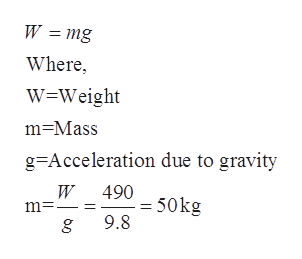# Sharon pushes a tall box across the floor. Her arms (and therefore her push force) of F = 400 N make a theta= 30 degree angle with the horizontal. Since the box rests on ball bearings it is essentially frictionless. Sick of her job, Sharon decides to give the box a brief shove lasting only t = 0.2 seconds. Find the velocity (magnitude and direction) of the box at the completion of Sharon's shove, assuming(a) the box's weight is 490 N, and(b) the box's weight is 122.5 N.In both cases, the box starts from rest.

Question
50 views

Sharon pushes a tall box across the floor. Her arms (and therefore her push force) of F = 400 N make a theta= 30 degree angle with the horizontal. Since the box rests on ball bearings it is essentially frictionless. Sick of her job, Sharon decides to give the box a brief shove lasting only t = 0.2 seconds. Find the velocity (magnitude and direction) of the box at the completion of Sharon's shove, assuming

(a) the box's weight is 490 N, and

(b) the box's weight is 122.5 N.

In both cases, the box starts from rest.

check_circle

star
star
star
star
star
1 Rating
Step 1

Given:

Step 2

Calculation:

Step 3

Part (a):

The weight of the ...help_outlineImage TranscriptioncloseW = mg Where W=Weight m-Mass g Acceleration due to gravity W 490 50kg m. = 9.8 g fullscreen

### Want to see the full answer?

See Solution

#### Want to see this answer and more?

Solutions are written by subject experts who are available 24/7. Questions are typically answered within 1 hour.*

See Solution
*Response times may vary by subject and question.
Tagged in

### Physics